﻿ How to Apply the Fundamental Parameters Method to the Quantitative X-ray Fluorescence Analysis of Geological Materials

### How to Apply the Fundamental Parameters Method to the Quantitative X-ray Fluorescence Analysis of Ge...

Richard RousseauOPEN ACCESSPEER-REVIEWED

## How to Apply the Fundamental Parameters Method to the Quantitative X-ray Fluorescence Analysis of Geological Materials

Richard RousseauLes logiciels R. Rousseau Inc., Cantley, Canada

### Abstract

The X-ray fluorescence (XRF) analysis of geological materials requires a robust analytical method because a large variety of elements to determine can be present in each sample and the concentration range of each one can be very large. In order to extract the maximum accuracy from the measured XRF intensities, a Fundamental Parameters (FP) method is proposed, but an adapted one to such an analytical context. The proposed adapted FP method consists of an appropriate sample preparation, a unique concentration calculation method and calibration procedure. It includes also a management of volatile components.

### At a glance: Figures

1
Prev Next

• Rousseau, Richard. "How to Apply the Fundamental Parameters Method to the Quantitative X-ray Fluorescence Analysis of Geological Materials." Journal of Geosciences and Geomatics 1.1 (2013): 1-7.
• Rousseau, R. (2013). How to Apply the Fundamental Parameters Method to the Quantitative X-ray Fluorescence Analysis of Geological Materials. Journal of Geosciences and Geomatics, 1(1), 1-7.
• Rousseau, Richard. "How to Apply the Fundamental Parameters Method to the Quantitative X-ray Fluorescence Analysis of Geological Materials." Journal of Geosciences and Geomatics 1, no. 1 (2013): 1-7.

 Import into BibTeX Import into EndNote Import into RefMan Import into RefWorks

### 1. Introduction

The quantitative analysis of any geological material is a real challenge. Indeed, it can be composed of almost all the elements of the periodic table, from Hydrogen to Uranium. The concentration of each element can be varying from a few ppms to 100%. Therefore, it takes a robust analytical method for obtaining the maximum accuracy from the measured XRF intensities when geological samples are analyzed.

The first step for developing a robust analytical method is to select a way to prepare the samples. The sample preparation must supply specimens that are completely homogeneous and have a perfectly flat and polished surface. Most importantly, the sample preparation must be reproducible. Also, the XRF spectrometer must be as stable as possible.

Then, the second important step is to select an algorithm for calculating sample compositions in association with an efficient calibration procedure. For the analysis of geological samples, the calibration procedure must be matrix independent and valid for the full analytical range, i.e. from 0 to 100%, of any element to determine (analyte). It must also include an efficient way to correct for instrumental drift in order to maintain the validity of the calibration data for a long period of time.

The selected algorithm for calculating the analyte concentrations must be able to correct accurately matrix effects. Currently, the FP method is included with the software supplied with each instrument. In this case, be aware that the theoretical relative error introduced by the algorithm alone should be less than 0.1%. Be aware also that the FP method has to be adapted to the analysis of geological samples. The following characteristics should be present:

1. Within a series of analytes, the user should be able to enter easily a mix of elements and oxides to be determined.

2. The FP method should be able to calibrate for large concentration ranges, from a few ppms to 100%, of any analyte.

3. The FP method should be able to take into account all the unmeasured components, for example, CO2, H2O, FeO, SO3, LOI, etc.

4. For the analysis of fused discs or pressed pellets, the FP method should be able to take into account the ratio sample/flux or binder.

5. For the analysis of fuse discs prepared with the original sample or the roasted one, the FP method should be able to calculate the sample composition with or without the knowledge of the LOI value.

This paper presents such a robust and adapted FP method.

### 2. Sample Preparation

The first step for developing a robust analytical method is to select a way to prepare the samples. To obtain the maximum accuracy from the measured XRF intensities the specimens must:

● Be perfectly homogeneous

● Have a flat and polished surface

● Have an infinite thickness

● And mainly the preparation of the specimen must be reproducible.

For applying all these characteristics to your specimens, it is recommended to prepare samples as fused discs.

### 3. KIM Equation

The second step for developing a robust analytical method is to select an algorithm for calculating sample compositions. The most general and basic expression used in XRF analysis for converting measured net intensities into concentrations is given by(1)

where

Ci　　Concentration of the analyte i in the sample

Ki　　Calibration factor

Ii　　Measured net intensity of i

Mis　Factor correcting the effect of the specimen matrix composition on the intensity of i

The K - I - M equation tells us that the calculated concentration of the analyte i (Ci) is equal to the product of three terms: first, the measured net intensity (Ii) corrected for matrix effects by the term Mis; and then, a calibration factor (Ki).

Let us see how to calculate these 3 terms.

3.1. Calculation of the Calibration Factor Ki

For a pure analyte specimen, Ci = 1, Mis = 1 and equation (1) becomes(2)

or(3)

where

I(i): Intensity measured from a specimen containing the pure analyte i

Equation (3) is telling us that the calibration factor is equal to the reciprocal of the net intensity of the pure analyte i. We will see how to use this feature in practice.

If we bring equation (3) into equation (1), we get(4)

If the relative intensity Ri is defined as being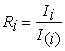(5)

This definition of the relative intensity Ri is very useful in quantitative XRF analysis. Indeed, if the measurement of intensities Ii and I(i) change with each spectrometer, their ratio Ri stays a constant, i.e. it is independent of the instrument. Ri is also independent of any instrumental drift with time.

If we bring equation (5) into equation (4), this last one becomes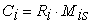(6)

or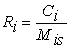(7)

When the relative intensities are measured, they are independent of the spectrometer specifications. They can also be calculated by the Sherman equation  or any algorithm in agreement with this equation, as for example, the Fundamental Algorithm .

3.2. Calculation of the Net Intensity Ii

In XRF analysis, the concentration is proportional to the net measured intensity. In this paper, I will not talk about instrumentation, operating conditions or how to measure X-ray fluorescence intensities. However, it is assumed that the measured intensities (Ii’) have been properly corrected for:

● background (Ibkg),

● line overlaps (fij)

● and blank (IB)

In geological samples, any analyte can be present at the level of trace or major. So, it is recommended to apply these corrections all the time. Translated in the mathematical language, it becomes:(8)

3.2.1. Background Subtractions

Because of the dilution, fused discs have a limited range of sample compositions and a limited degree of background curvature. In this case, the following method for background subtraction is proposed. By measuring on a blank specimen a background (bkg) free of peaks and the bkg beneath the analytical peak, the ratio between these two bkg measurements can be calculated. For any other specimen, the bkg values at the same positions will change, but their ratio will stay the same . Thus, knowing the ratio value and by measuring the same bkg free of peaks on any other specimen, we can calculate the bkg beneath the analytical peak. Figure 1 shows an example of this type of background calculations.

The advantage of this bkg subtraction method is that only one bkg free of peaks has to be measured for each analytical peak. This measurement can even be used for multiple analytical peaks as shown by Figure 1. Also, if necessary, the ratio value of a given analyte can be adjusted by making the intercept of its calibration line equal to zero.

Figure 1. By measuring on a blank specimen a bkg free of peaks and the bkg at peak positions, we can calculate their ratios. For fused discs, these ratios can be used to calculate bkg at peak positions for any other specimen.

3.2.2. Spectral Interferences

To determine the spectral interference of one element on another, prepare 4 specimens containing different concentrations of the interfering element j and no detectable amount of the overlapped element i. Measure the net intensity of the interfering element j and the contribution of this element in position of the overlapped element i. Plot both intensities. The slope is the interference factor fij. Figure 2 shows an example of the calculation of the factor fij in the case of Ba-Ti.

Figure 2. Plot of the net intensity of the interfering element Ti versus the contribution of this one in position of the overlapped element Ba. The measurements have been done on 4 specimens containing different amount of the interfering element Ti, but no amount of the overlapped element Ba. The slope of the line is equal to the interference factor fBaTi
3.3. Calculation of the Mis Factor Correcting for Matrix Effects

The Mis factor corrects for the effect of the matrix composition of the specimen “s” on the measured XRF intensity of the analyte i.

At the beginning of the seventies, many algorithms had been proposed to correct for matrix effects. But, which one to choose for the analysis of geological samples?

All of them have been tested, including the FP method. All of them had their strengths and weaknesses, but most of the time, they were approximation designed for specific applications. None was valid for calculating large concentration ranges of any analyte, in any type of sample compositions.

Therefore, there is a need for a new algorithm, as universal, as general as possible, able to calculate as accurately as possible the composition of any geological sample. In other words, is there a fundamental algorithm from which one can deduce all the other proposed algorithms ? Is there a fundamental algorithm which would be the synthesis of all the knowledge accumulated since the fifties when a specimen is bombarded by an X-ray beam?

For the analysis of geological samples, a more general, universal, or fundamental algorithm (FA) is then proposed , which is: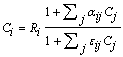(9)

where the influence coefficients αij and εij correct respectively for the absorption and enhancement effects of each matrix element j on the analyte i.

All the mathematical details of this fundamental algorithm [4, 5] will not be explained here, neither its powerful properties , but keep in mind that the FA has been deduced from the Sherman equation in an exact manner, without doing any approximation, taking care to respect rigorously the fundamental Sherman equation in every respect: algebraically, mathematically and physically .

The Mis factor can be calculated by the Sherman equation  or any other algorithm in agreement with this equation, as for example, the Fundamental Algorithm .

### 4. Calibration with a Single Standard

In 1968, Criss and Birks proposed the Fundamental Parameters (FP) method  to calculate the composition of a sample analysed by XRF. Their method is as follows.

As a specimen of the pure analyte i is not always available, it is better to rewrite the equation (4) as follows:(10)

or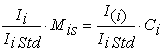(11)

where Ii Std is the measured net intensity of the analyte i in a selected standard similar to the unknown samples to be analysed.

The equation (11) has the general form of a straight line:If we plot the calibration line of the Ii Mis/Ii Std ratio (Y axis) as a function of Ci (X axis), the calculated slope mi of the line is equivalent to the ratio I(i)/Ii Std.

In the equation (11), the ratio on the left is measured, the Mis factor is calculated by the Sherman equation from the composition of the selected standard and Ci is the concentration of the analyte i in the standard. The ratio on the right is equal to the slope of the calibration line. Thus, we can calibrate with a single standard similar to the samples to be analysed.

This way of calibrate has two great advantages. First, as the theory is imperfect, we need a scaling factor in order to adapt theory to the experimental data of each spectrometer, knowing very well that theory cannot account for all variations in all the instrumental parameters. This factor is the slope of the calibration line, which represents the intensity I(i) of the pure analyte. The second great advantage is that we do not have to measure the intensity of any pure analyte.

After the calibration, the slope mi is known and rearranging the equation (11), each concentration Ci of the unknown samples is calculated with(12)

In this equation, the Mis factor is calculated with the Sherman equation. It has to be calculated by iteration for each analyte i in each unknown sample. As Mis depends of the composition of the unknown sample, we have to calculate a first estimate of the composition as follows:

If(13)

then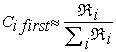(14)

where Ci first is the first estimate of the concentration i in the unknown sample calculated from the measured intensities without any matrix effect correction. With the equation (14), it is assumed that the first estimate of the sample composition is equal to the measured relative intensities normalized to 100%. This approach will produce accurate results if, and only if, the intensities of all the major and minor elements are measured and if the composition of the reference standard is very similar to the one of unknown samples.

This method is perfect for the analysis of samples having very short concentration ranges and when a standard similar to the unknown samples can be found easily, as for example, steels. In this case, the Sherman equation converges all the time and gives accurate results. For geological materials, the analytical context is different because the concentration ranges are very large and a large variety of standards are then necessary to cover all the unknown compositions. Here a solution to solve these problems.

### 5. Calibration with a Few Standards

In 1982, Rousseau proposed the Fundamental Algorithm to extend the application of the FP method [7, 8]. Here a summary of his approach.

The equation (4) can be rewritten as follows: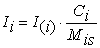(15)

Dividing on both sides by IiM, the measured gross intensity of the analyte i in a drift monitor, gives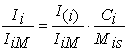(16)

Again, we have the equation of a straight line having the general form(17)

If we plot the calibration line of the Ii/IiM ratio (Y axis) as a function of Ci/Mis (X axis), the calculated slope mi of the line is equal to the ratio I(i)/IiM.

The combination of equations (7), (16) and (17) leads to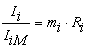(18)

Thus, the calibration procedure compares the measured relative intensity (Ii/IiM) to the calculated one (Ri). This simple, but at the same time, very efficient strategy allows comparing measured and calculated relative intensities of any standard, no matter their composition. In this case, the calibration line is said matrix independent, which is a strategy perfectly adapted to the analysis of any geological material.

Equation (16) is the equation of a straight line without intercept. However, we can add an intercept bi to take into account the subtraction of an incorrect background or overlap values from the measured gross intensity. If in addition, the ratio I(i)/IiM is replaced by the slope mi of the calibration line, we get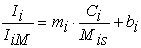(19)

Equation (19) is the one to use to perform the calibration of any analyte in any geological material. Figure 3 shows an example of the type of calibration straight line represented by this equation.

Figure 3. Fe calibration graph using NIST alloy standards. The graph of measured relative intensities as a function of the concentration CFe gives scattered points (o). On the other hand, the plot of the same measured relative intensities as a function of the theoretical relative intensities calculated by the Sherman equation lines up each point (•) on the calibration line

This calibration procedure offers many great advantages. First, it compares the measured relative intensities to the calculated ones. By so doing, the slope becomes a scaling factor allowing adapting theory to the experimental data of each XRF spectrometer, knowing very well that theory cannot account for all variations in all the instrumental parameters. This powerful feature allows extracting the maximum accuracy from the measured XRF intensities.

Also, the calibration slope is equivalent tot the XRF intensity of the pure analyte [I(i)], which means that it is not necessary to have to measure any pure analyte specimen.

Furthermore, it is matrix independent, which means that the standard compositions do not have to be similar to the unknown ones.

Finally, if the intensities Ii and IiM change with time further to the instrumental drift, their ratio remains constant. This features guarantees that your calibration data will stay valid for a long period of time, which contribute to maintain the accuracy of your results.

### 6. Calculation of Concentrations

For calculating the concentration Ci, the equation (19) can be rearranged as: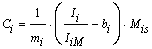(20)

This equation shows us that if there are some errors in the background calculation, bi is different from zero and subtracted from the measured net intensity Ii before being corrected for matrix effects by the Mis factor. In addition, the intensity Ii is corrected for the instrumental drift by the intensity IiM. Finally, the calibration slope mi takes into account the imperfections of theory by adapting it to the measured data of each spectrometer. We can say then that this equation makes physical sense.

The composition of an unknown sample is calculated by applying an iteration process to the equation (20). Again, as the Mis factor depends of the composition of the unknown sample, we have to calculate a first estimate of the composition as follows:

If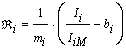(21)

Then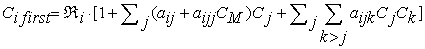(22)

where Ci first is the first estimate of the concentration i in the unknown sample calculated from the measured intensities modified by the Claisse-Quintin (CQ) algorithm to correct for matrix effects . The influence coefficients aij, aijj and aijk are constant calculated for a representative sample. The matrix concentration CM = 1 – Ci. Once that the first estimate of the composition is calculated, it is used to calculate once the influence coefficients αij and εij within the Fundamental Algorithm:(23)

The final composition is calculated by applying an iterative process to this algorithm, where all the coefficients αij and εij are kept constant.

It is necessary to use the CQ algorithm to calculate the first estimate of the sample composition because it insures that the first estimate composition will be quite close to the final composition and makes equation (23) converges all the time with only a few iterations. Otherwise, the first estimate of the composition can be very different of the final one and we have no guarantee that the equation (23) will converge toward the true final composition.

This last complete method can be applied using the CiROU software written by the author.

If the concentration ranges of the unknown samples are smaller than 10%, as in the case of the determination of oxides in rock samples diluted in fused discs, the equation (23) can be replaced by(24)

where the influence coefficient aij is a constant value for a series of unknown samples calculated from a representative composition by the following equation: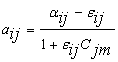(25)

where the coefficients αij and εij from the Fundamental Algorithm are calculated for the special case of a binary standard having a composition (Cim, Cjm) and where Cim is the mid-value of the calibration range of the analyte i. The Cjm concentration is calculated from(26)

This last approach introduces some approximations, but the calculation method by itself (Eqns 24, 25 and 26) introduces a theoretical mean relative error of only 0.02% on calculated concentrations when applied to concentration ranges smaller than 10% . This last complete method can be applied using the CiLT software written by the author.

### 7. Management of Volatile Components

For getting the maximum accuracy, geological samples are prepared as fused disc. During the preparation, some volatile components (CO2, H2O, …) can be lost. Thus, the ratio sample/flux is modified and can affect severely the results. I am proposing 3 solutions to solve this problem.

The first analytical context and solution are as follows:

1. The sample is burned, the LOI value is known and the roasted sample is used to prepare the fused disc.

As example, let us consider the following sample composition. Let us assume that there are 10% of volatile components:

25% + 20% + 15% + 30% + 10% = 100%

25% + 20% + 15% + 30% = 100% - 10%

25% + 20% + 15% + 30% = 90%

27.8% + 22.2% + 16.7% + 33.3% = 100%

As we do LOI before the fusion, there is no loss during the fusion and the last composition is the new one of the sample to analyze. After the calculation of the concentrations with the equation (27), we have to reduce the concentrations with the factor (1-LOI) to bring them to their original values.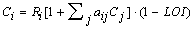(27)

The second analytical context and solution are as follows:

The sample is burned, the LOI value is known and the original sample is used to prepare the fused disc.

In this case, there is a loss of volatiles components during the fusion, the sample/flux ratio varies and the concentrations in the specimen increase. In this case, they are reduced by adding a giv coefficient to the equation (28), which is always a negative value and where Cv = LOI.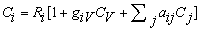(28)

The coefficient giv is calculated from the Sherman equation and takes into account the change of the sample/flux ratio due to the loss of volatile components. Presently, the calculation of the giv coefficient is confidential.

The third analytical context and solution are as follows:

3. The LOI value is unknown and the original sample is used to prepare the fused disc.

In this case, the LOI value is unknown and the concentrations are calculated by the equation (29), where the Cv concentration term has been suppressed.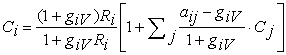(29)

Then, the Cv concentration is calculated by difference with the following equation:(30)

Once the Cv concentration is known, the final concentrations are calculated with the equation (28).

### 8. Experimental Results

Let us see now some practical results obtained with the proposed method. After having analyzed more than 160,000 geological samples on a period of more than 36 years at the Geological Survey of Canada, I can say that the accuracy of results remains unsurpassed. See some calculated compositions of geological samples at Table 1.

#### Table 1. List of some sample compositions calculated with the proposed methodDownload asPowerPoint Slide

Veiw figureView current table in a new window

In these samples, the concentration of SiO2 varies from 22% to 98%. In spite of that, the total of the calculated compositions is always equal to 100% ± 1% without doing any normalization. For an XRF analyst, it is a real satisfaction to get such accurate results, day after day, sample after sample, no matter their compositions.

### 9. Conclusion

The proposed method is the synthesis of the best features of all the methods developed during 30 years, from 1955 to 1985.

It allows getting the maximum accuracy from the measured XRF intensities because of its unique calculation method, calibration procedure and the management of volatile components.

It is valid for large concentration ranges of diluted geological samples.

The accuracy of results depends only on the quality of sample preparation and the standards used.

### References

  Sherman J. The theoretical derivation of fluorescent X-ray intensities from mixtures. Spectrochimica Acta. 1955; 7: 283-306.In article  Rousseau R.M. The quest for a Fundamental Algorithm in X-ray fluorescence analysis and calibration. The Open Spectroscopy Journal. 2009; 3: 31-42.In article CrossRef  Willis J.P. and Duncan A.R. Understanding XRF Spectrometry, Volume 2: Quantitative analysis and special sample preparation and presentation methods. Almelo: PANalytical B.V. 2008; 13-11 to 13-16.In article  Rousseau R.M. and Boivin J.A. The Fundamental Algorithm: a natural extension of the Sherman equation, part I: theory. The Rigaku Journal. 1998; 15 (1): 13-15.In article  Rousseau R.M. Corrections for matrix effects in X-ray fluorescence analysis-A tutorial. Spectrochimica Acta Part B. 2006; 759-777.In article  Criss J.W. and Birks L.S. Calculation methods for fluorescent X-ray spectrometry, empirical coefficients vs. fundamental parameters. Analytical Chemistry. 1968; 40 (7): 1080-1087.In article CrossRef  Rousseau R.M. Fundamental algorithm between concentration and intensity in XRF analysis, Part 1: theory. X-Ray Spectrom. 1984; 13 (3): 115-120.In article CrossRef  Rousseau R.M. Fundamental Algorithm between concentration and intensity in XRF analysis, part 2: practical application. X-Ray Spectrom. 1984; 13 (3): 121-125.In article CrossRef  Rousseau R.M. Concept of the influence coefficient. The Rigaku Journal. 2001; 18 (1): 8-21.In article